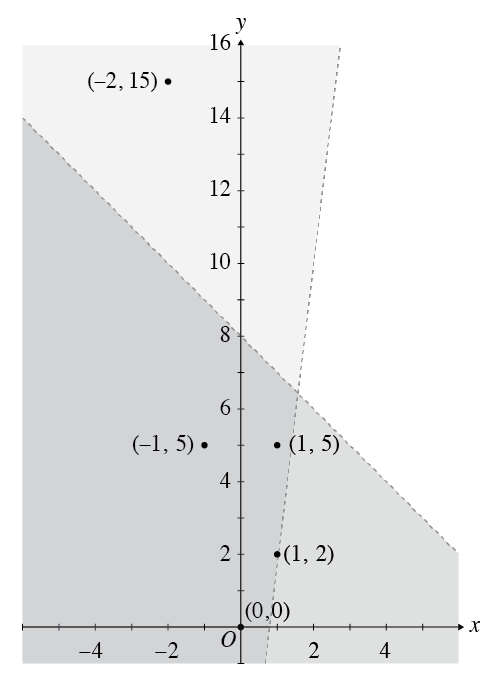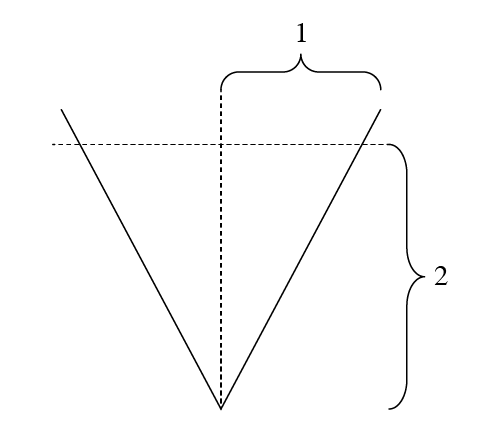## 1.

The Correct Answer is (C) — Subtract 10 from both sides to get 3x–3 = 9. From here you can see that since 32 = 9, x – 3 = 2, so x = 5.

## 2.

The Correct Answer is (C) — Of the possible factors given, only 10 is a product of the prime factors listed in the question. The number 28,210 is an example of a number that has the listed prime factors but is not divisible by 4, 6, 15, or 25.

## 3.

The Correct Answer is (A) — Slope is calculated as “rise” (change in y-value) divided by “run” (change in x-value). In this case, the rise is 4 – 3 = 1 and the run is 5 – (–2) = 7, so the slope is 17. As a decimal, this fraction is approximately 0.14.

If you chose (A) you may have subtracted the y-values from the x-values.
If you chose (E) you may have divided run by rise.
If you chose (D) you may have done both these things.

## 4.

The Correct Answer is (D) — To find the magnitude of a vector, square each component, add them together, and then take the square root. Here is how we find the magnitude in this case: $$\sqrt{5^2+0^2+7^2}=\sqrt{74}=8.6$$.

## 5.

The Correct Answer is (A) — This question hinges on your understanding of exponent rules. A positive exponent in a denominator is equivalent to a negative exponent in a numerator, and when multiplying two terms with the same base, simply add the exponents. With these rules in mind, first simplify the fraction: $$\frac{a^{-b}}{a^{a}} = a^{-b} a^{-a}$$ or $$a^{-a-b}$$ Next, we multiply by $$a^2$$ (that is, add the exponents) to get: $$a^{(-a-b+2)}$$

If you chose (B), you may have forgotten the rules for exponents in denominators.

## 6.

The Correct Answer is (A) — A linear function with nonzero slope will be either continuously increasing or continuously decreasing, meaning that no two $x$-values share a $y$-value (if they did, the function would either be nonlinear or its slope would be zero).

(B) is incorrect because two different $x$-values must correspond to two different $y$-values.

(C) and (D) are incorrect because even though one of them must be false, it is impossible to know which one without more information (answer (C) would be true if the function’s slope was negative; answer (D) if the slope was positive).

(E) is incorrect because we know nothing about the function’s $x$-intercepts.

## 7.

The Correct Answer is (E) — You can draw a right-angled triangle and label one of the acute angles θ. You can label the side opposite θ as having length 4, and the hypotenuse as having length 5, since you know that sin⁡ θ = 45. You can use the Pythagorean Theorem to find that the remaining side of the triangle has length 3, and since cosine is equal to adjacent divided by hypotenuse, you know that cos⁡ θ = 35.

## 8.

The Correct Answer is (D) — The function is undefined at any $x$-value that makes its denominator zero, since dividing by zero is undefined. While you can plug in the given answer choices, it is much faster to factor the denominator, which leaves you with the fraction $\frac{7}{x(x-2)(x+3)(x-6)}$ . The values of $x$ that make this denominator equal to 0 are 0, 2, –3, and 6. The only answer choice that is not one of these numbers is 3.

## 9.

The Correct Answer is (B) — We are given a statement of the form “If P then Q”. Any statement of this form is equivalent to the statement “If not-Q then not-P”, since if Q is false, then P cannot be true because P being true would make Q true as well—so P must be false. Statements such as “If Q then P” and “It not-P then not-Q” are not equivalent to this statement.

Instead, you can use your knowledge of numbers to find which statement is true. (A) is false if $a=b=1$. (C) and (D) are also false when $a=b=1$. (E) is false when $a=1$ and $b=2$, for example.

## 10.

The Correct Answer is (B) — If the mean of 7 numbers is 15, their sum must be 7 × 15 = 105. Similarly, if the mean of 8 numbers is 12, their sum must be 8 × 12 = 96. The eighth number must account for the difference between these two sums: 96 – 105 = –9.

## 11.

The Correct Answer is (C) — When evaluating the composition of various functions, you should work from the inside out. Start by plugging h (x) into g (x) to get g (h (x)) = 5(1 – x2) + 3 = –5x2 + 8. Now, plug your result into f (x) to find the answer: 3(–5x2 + 8) = –15x2 + 24. If you got (D) or (E), you may have tried to multiply the functions instead of substituting them into one another.

## 12.

The Correct Answer is (D) — Remember that a valid function of x should pass a “vertical line test,” meaning it assigns only one output to each input, or only one possible y-value for every x-value. Answer choice (D) fails the vertical line test: for example, at x = 0, there are two possible values for y.

## 13.

The Correct Answer is (E) — A line is perpendicular to another line if its slope is the negative reciprocal of the first line, and vice versa. The line y = ½ x – 3 has a slope of ½, so a line with a slope of –2 would be perpendicular to it. (E) is the only answer option with this slope.

If you got (A), you found the negative slope but forgot to take the reciprocal.
If you got (C), you took the reciprocal of the entire line equation.
If you got (D), you took the reciprocal of the slope but forgot to change the sign.

## 14.

The Correct Answer is (E) — A reflection across the line y = x is the same thing as inverting the y and x values (for example, the point $(3,4)$ gets reflected to the point $(4,3)$). If pentagon P has vertices at $(–2, –4)$, $(–4, 1)$, $(–1, 4)$, $(2, 4)$, and $(3, 0)$, the new pentagon would have vertices at $(–4, –2)$, $(1, –4)$, $(4, –1)$, $(4, 2)$, and $(0, 3)$. Only one of these vertices is an answer choice: $(–4, –2)$.

## 15.

The Correct Answer is (A) — Seeing that the answers are all inverse trigonometric functions should alert you to the fact that you can create a right-angled triangle from the information in the question, with the hypotenuse going between the tops of the two poles. The poles are 12 meters apart, so the bottom side of the triangle has length 12. One is 5 meters taller, so the leg opposite to the angle of elevation has length 5. The tangent of the angle of elevation is equal to , so the angle of elevation itself is $\tan^{-1}$ .

## 16.

The Correct Answer is (A) — You can use the sine law to isolate and solve for x:

If you chose (A), you found x, not sin (x).
If you chose (E), you may have thought that the a’s wouldn’t cancel out.

## 17.

The Correct Answer is (A) — The easiest way to solve this question is to graph both inequalities to get a sense of the solution space. You are looking for a point that is either lower than the line $y = 9x – 8$ or higher than the line $y = –x + 8$.The only point not strictly in the shaded area is $(–2, 15)$, which is above the line $y= –x + 8$. Therefore, it is not a solution to the inequalities and the correct answer is (A).

## 45.

The Correct Answer is (C) — To find the point or points where a graph crosses its oblique asymptote, set the function equal to the asymptote and solve for x (the asymptote is y = x + 5, the polynomial part of the function):

If you got (D) or (E), you were trying to set the entire function equal to zero.

## 46.

The Correct Answer is (B) — The easiest way to answer this question is to graph the given equations on your graphing calculator, remembering that 0 ≤ t ≤ π.

If you mistakenly include the values π ≤ t ≤ 2π, you will get a complete circle, or answer choice (A).

## 47.

The Correct Answer is (D) — The easiest way to solve this problem is to move the lines off the coordinate plane and treat it as a geometry problem. Because you know the slopes of the lines are 2 and –2, you can add in a horizontal and vertical line and label your diagram with these measurements:Each of the smaller angles at the bottom has measure $$\frac{\theta}{2}$$. From the diagram, you know that $$\frac{\theta}{2} = \tan ^{-1} \left(\frac12 \right ) = 26.565°$$, so $$\theta = 53.13°$$.

## 48.

The Correct Answer is (C) — The four possible outcomes are rain and sun, rain and no sun, no rain and sun, and no rain and no sun. The probabilities for these four options must add up to 1, since there are no other options. The question tells you that the probability of rain and no sun is 0.3. It also tells you that the probability of no rain, which is equal to the probability of no rain and sun plus the probability of no rain and no sun, is 0.4. This means that three of the four options combined have a 0.7 probability of happening, meaning that the fourth option, rain and sun, has a probability of 0.3 of occurring.

## 49.

The Correct Answer is (A) — You can plug the given numbers into the equation to get 750 = 100er5. Divide both sides by 100 to get 7.5 = er5, and then take the natural logarithm of both sides: 5r = ln(7.5) ≈ 2.015. Divide by 5 to get r ≈ 0.403.

## 50.

The Correct Answer is (B) — If the radius of the sphere is 8, its diameter is 16. Since opposite vertices of the cube lie on the diameter of the sphere, the distance between opposite vertices is also 16. You can use the distance formula to find the side length of the cube; if a is the side length, then 162 = a2 + a2 + a2 = 3a2, so a = = 9.2376. Since the area of any face of the cube is equal to a2, the surface area of the entire cube is equal to 6a2 = 6 × 9.23762 = 512.00.